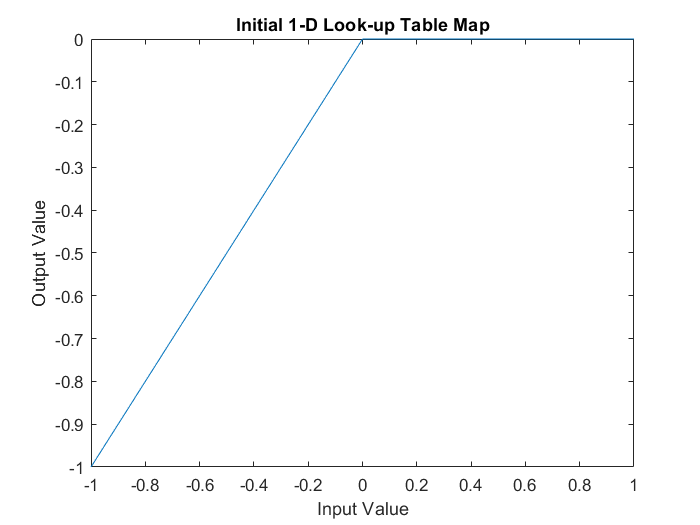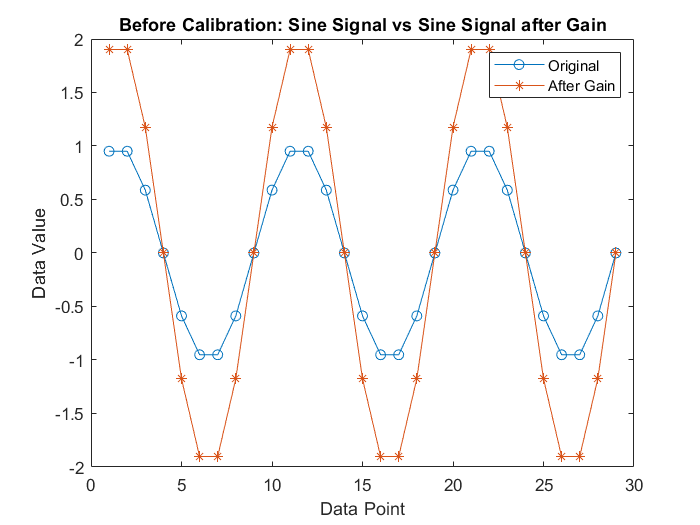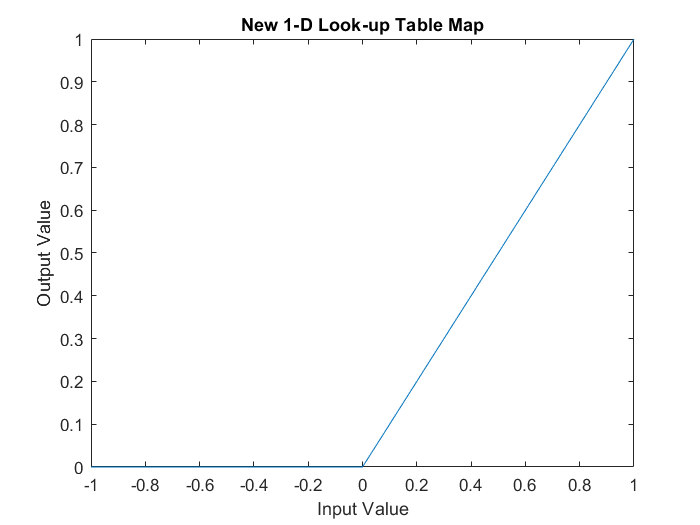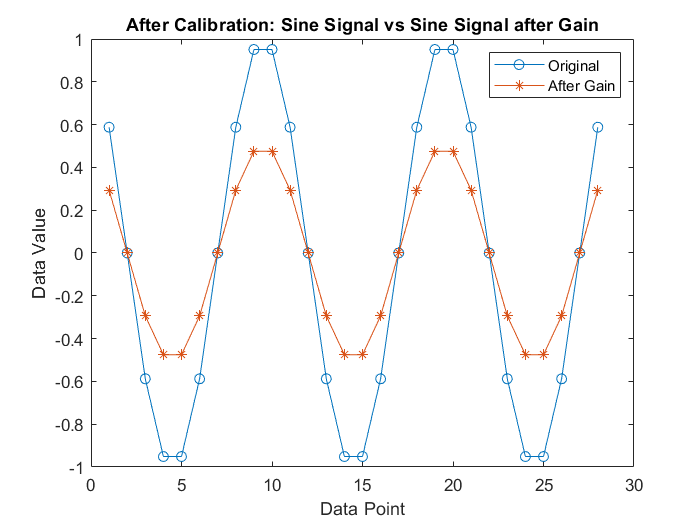# Calibrate XCP Characteristics

This example shows how to use the XCP protocol capability to connect and calibrate available characteristic data from a Simulink model deployed to a Windows executable. The example writes to modify the parameters of the model using TCP and direct memory access, and compares the measurements before and after calibration. XCP is a high-level protocol used for accessing and modifying internal parameters and variables of a model, algorithm, or ECU. For more information, refer to the ASAM standards.

### Algorithm Overview

The algorithm used in this example is a Simulink model built and deployed as an XCP server. The model has already been compiled and is available to run in the file `XCPServerSineWaveGenerator.exe`. Additionally, the A2L-file `XCPServerSineWaveGenerator.a2l` is provided as an output of that build process. The model contains three measurements and two characteristics, accessible via XCP. Because the model is already deployed, Simulink is not required to run this example. The following image illustrates the model.The signal `SineAfterGain` is obtained by using the multiplier `Gain` to scale the source signal `Sine`, and the signal `SineAfterTable` is obtained by using the 1-D look-up table to modify the source signal `Sine`. Calibrating the parameter `Gain` and the 1-D look-up table produces different `SineAfterGain` and `SineAfterTable` waveforms, correspondingly.

For details about how to build a Simulink model, including an XCP server and generating an A2L-file, see Export ASAP2 File for Data Measurement and Calibration (Simulink Coder).

### Run the XCP Server Model

To communicate with the XCP server, the deployed model must be run. By using the `system` function, you can execute the `XCPServer.exe` from inside MATLAB. The function requires constructing an argument list pointing to the executable. A separate command window opens and shows running outputs from the server.

```sysCommand = ['"', fullfile(pwd, 'XCPServerSineWaveGenerator.exe'),'"', ' &']; system(sysCommand);```

### Open the A2L-File

An A2L-file is required to establish a connection to the XCP server. The A2L-file describes all the functionality and capability that the XCP server provides, as well as the details of how to connect to the server. Use the `xcpA2L` function to open the A2L-file that describes the server model.

`a2lInfo = xcpA2L("XCPServerSineWaveGenerator.a2l")`
```a2lInfo = A2L with properties: File Details FileName: 'XCPServerSineWaveGenerator.a2l' FilePath: 'C:\Users\siyingl\OneDrive - MathWorks\Documents\MATLAB\Examples\vnt-ex09112476\XCPServerSineWaveGenerator.a2l' ServerName: 'ModuleName' Warnings: [0×0 string] Parameter Details Events: {'100 ms'} EventInfo: [1×1 xcp.a2l.Event] Measurements: {'Sine' 'SineAfterGain' 'SineAfterTable' 'XCPServer_DW.lastCos' 'XCPServer_DW.lastSin' 'XCPServer_DW.systemEnable'} MeasurementInfo: [6×1 containers.Map] Characteristics: {'Gain' 'ydata'} CharacteristicInfo: [2×1 containers.Map] AxisInfo: [1×1 containers.Map] RecordLayouts: [4×1 containers.Map] CompuMethods: [3×1 containers.Map] CompuTabs: [0×1 containers.Map] CompuVTabs: [0×1 containers.Map] XCP Protocol Details ProtocolLayerInfo: [1×1 xcp.a2l.ProtocolLayer] DAQInfo: [1×1 xcp.a2l.DAQ] TransportLayerCANInfo: [0×0 xcp.a2l.XCPonCAN] TransportLayerUDPInfo: [0×0 xcp.a2l.XCPonIP] TransportLayerTCPInfo: [1×1 xcp.a2l.XCPonIP] ```

TCP is the transport protocol used to communicate with the XCP server. Details for the TCP connection, such as the IP address and port number, are contained in the `TransportLayerTCPInfo` property.

`a2lInfo.TransportLayerTCPInfo`
```ans = XCPonIP with properties: CommonParameters: [1×1 xcp.a2l.CommonParameters] TransportLayerInstance: '' Port: 17725 Address: 2.1307e+09 AddressString: '127.0.0.1' ```

### Create an XCP Channel

To create an active XCP connection to the server, use the `xcpChannel` function. The function requires a reference to the server A2L-file and the type of transport protocol to use for messaging with the server.

`xcpCh = xcpChannel(a2lInfo, "TCP")`
```xcpCh = Channel with properties: ServerName: 'ModuleName' A2LFileName: 'XCPServerSineWaveGenerator.a2l' TransportLayer: 'TCP' TransportLayerDevice: [1×1 struct] SeedKeyDLL: [] ```

### Connect to the Server

To make communication with the server active, use the `connect` function.

`connect(xcpCh)`

### View Available Characteristics from A2L-File

A characteristic in XCP represents a tunable parameter in the memory of the model. Characteristics available for calibration are defined in the A2L-file and can be found in the `Characteristics` property. Note that the parameter `Gain` is the multiplier and `ydata` specifies the output data points of the 1-D look-up table.

`a2lInfo.Characteristics`
```ans = 1×2 cell {'Gain'} {'ydata'} ```
`a2lInfo.CharacteristicInfo("Gain")`
```ans = Characteristic with properties: Name: 'Gain' LongIdentifier: '' CharacteristicType: VALUE ECUAddress: 549960 Deposit: [1×1 xcp.a2l.RecordLayout] MaxDiff: 0 Conversion: [1×1 xcp.a2l.CompuMethod] LowerLimit: -5 UpperLimit: 5 Dimension: 1 AxisConversion: {1×0 cell} BitMask: [] ByteOrder: MSB_LAST Discrete: [] ECUAddressExtension: 0 Format: '' Number: [] PhysUnit: '' ```
`a2lInfo.CharacteristicInfo("ydata")`
```ans = Characteristic with properties: Name: 'ydata' LongIdentifier: 'Y data' CharacteristicType: CURVE ECUAddress: 550024 Deposit: [1×1 xcp.a2l.RecordLayout] MaxDiff: 0 Conversion: [1×1 xcp.a2l.CompuMethod] LowerLimit: -2 UpperLimit: 2 Dimension: 7 AxisConversion: {[1×1 xcp.a2l.CompuMethod]} BitMask: [] ByteOrder: MSB_LAST Discrete: [] ECUAddressExtension: 0 Format: '' Number: [] PhysUnit: '' ```

Read the current value of the characteristic `Gain`. The `readCharacteristic` function performs a direct read from the server for a given characteristic.

`initialGain = readCharacteristic(xcpCh, "Gain")`
```initialGain = 2 ```

Read the current 1-D look-up table characteristic using `readAxis` and `readCharacteristic`, then plot the mapping. This table effectively maps any positive input value to zero output.

`inputBreakpoints = readAxis(xcpCh, "xdata")`
```inputBreakpoints = 1×7 -1.0000 -0.5000 -0.2000 0 0.2000 0.5000 1.0000 ```
`outputPoints = readCharacteristic(xcpCh, "ydata")`
```outputPoints = 1×7 -1.0000 -0.5000 -0.2000 0 0 0 0 ```
```plot(inputBreakpoints, outputPoints); title("Initial 1-D Look-up Table Map"); xlabel("Input Value"); ylabel("Output Value");```### Create a Measurement List

This example explores the value of the measurement `Sine`, unmodified and modified by the two characteristics. To visualize the continuously changing value of `Sine` pre- and post-calibration, acquire measurement data values using a DAQ list. Use the `createMeasurementList` function to create a DAQ list containing all `Sine`-based measurements available from the server.

`createMeasurementList(xcpCh, "DAQ", "100 ms", ["Sine", "SineAfterGain", "SineAfterTable"])`

### Obtain Measurements Before Calibration

Use the `startMeasurement` function and `stopMeasurement` function to run the DAQ list for a short period of time.

```startMeasurement(xcpCh); pause(3); stopMeasurement(xcpCh);```

To retrieve the data acquired by the DAQ list for all the Sine-based measurements, use the `readDAQ` function. The number of retrieved samples during 3 seconds at 100 ms event is expected to be 30, but because the XCP server runs on Windows, which is not a real-time operating system, the actual number of retrieved samples might be less than 30, depending on how occupied the operating system is.

```sine = readDAQ(xcpCh, "Sine"); sineAfterGain = readDAQ(xcpCh, "SineAfterGain"); sineAfterTable = readDAQ(xcpCh, "SineAfterTable");```

### Inspect Measurements Before Calibration

Plot the `SineAfterGain` measurement against the base `Sine` measurement. The value after `Gain` is boosted by a factor of 2, based on the original measurement, because the preloaded value of the characteristic `Gain` is 2, as shown previously.

```plot(sine, "o-"); hold on; plot(sineAfterGain, "*-"); hold off; title("Before Calibration: Sine Signal vs Sine Signal after Gain"); legend("Original", "After Gain"); xlabel("Data Point"); ylabel("Data Value");```Plot the `SineAfterTable` measurement against the base `Sine` measurement. Any positive value of the original measurement is mapped to zero according to the preloaded 1-D look-up table, therefore the modified signal looks truncated and does not have any positive values.

```plot(sine, "o-"); hold on; plot(sineAfterTable, "*-"); hold off; title("Before Calibration: Sine Signal vs Sine Signal after Table"); legend("Original", "After Table"); xlabel("Data Point"); ylabel("Data Value");```### Calibrate the Gain and 1-D Look-up Table

Write a new value to the charateristic `Gain` using `writeCharacteristic`, and perform a read to verify the change using `readCharacteristic`.

```writeCharacteristic(xcpCh, "Gain", 0.5); newGain = readCharacteristic(xcpCh, "Gain")```
```newGain = 0.5000 ```

Write new data points to the output of the 1-D look-up table using `writeCharacteristic`.

`writeCharacteristic(xcpCh, "ydata", [0 0 0 0 0.2 0.5 1]);`

Read the new 1-D look-up table data using `readAxis` and `readCharacteristic`, then plot the mapping. Now the table effectively maps any negative input value to zero output.

`inputBreakpoints = readAxis(xcpCh, "xdata")`
```inputBreakpoints = 1×7 -1.0000 -0.5000 -0.2000 0 0.2000 0.5000 1.0000 ```
`newOutputPoints = readCharacteristic(xcpCh, "ydata")`
```newOutputPoints = 1×7 0 0 0 0 0.2000 0.5000 1.0000 ```
```plot(inputBreakpoints, newOutputPoints); title("New 1-D Look-up Table Map"); xlabel("Input Value"); ylabel("Output Value");```### Obtain Measurements after Calibration

Use the `startMeasurement` function and `stopMeasurement` function to run the DAQ list for a short period of time.

```startMeasurement(xcpCh); pause(3); stopMeasurement(xcpCh);```

To retrieve the data acquired by the DAQ list for all the Sine-based measurements, use the `readDAQ` function.

```sine = readDAQ(xcpCh, "Sine"); sineAfterGain = readDAQ(xcpCh, "SineAfterGain"); sineAfterTable = readDAQ(xcpCh, "SineAfterTable");```

### Inspect Measurements After Calibration

Plot the `SineAfterGain` measurement against the base `Sine` measurement. Now the value after `Gain` is decreased by a factor of 2, based on the original measurement, because the value of the characteristic `Gain` is set to 0.5 after calibration.

```plot(sine, "o-"); hold on; plot(sineAfterGain, "*-"); hold off; title("After Calibration: Sine Signal vs Sine Signal after Gain"); legend("Original", "After Gain"); xlabel("Data Point"); ylabel("Data Value");```Plot the `SineAfterTable` measurement against the base `Sine` measurement. Any negative value of the original measurement is mapped to zero according to the new 1-D look-up table, therefore the modified signal looks truncated differently and does not have any negative values.

```plot(sine, "o-"); hold on; plot(sineAfterTable, "*-"); hold off; title("After Calibration: Sine Signal vs Sine Signal after Table"); legend("Original", "After Table"); xlabel("Data Point"); ylabel("Data Value");```### Disconnect from the Server

To deactivate communication with the server, use the `disconnect` function. The XCP server can be safely closed after disconnecting.

`disconnect(xcpCh)`

### Clean Up

`clear a2lInfo`

## SupportGet trial now